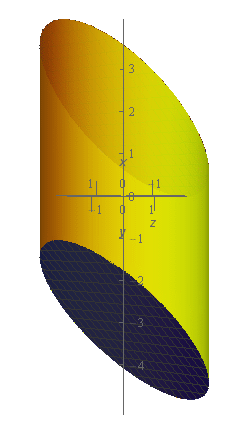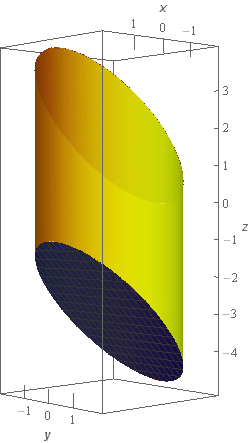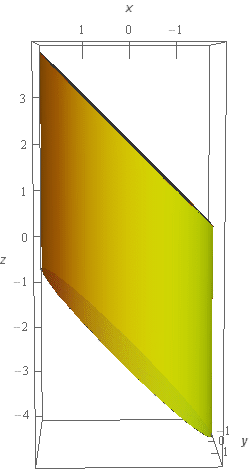Paul's Online Notes
Home / Calculus III / Surface Integrals / Surface Integrals of Vector Fields
Show Mobile Notice Show All Notes Hide All Notes
Mobile Notice
You appear to be on a device with a "narrow" screen width (i.e. you are probably on a mobile phone). Due to the nature of the mathematics on this site it is best views in landscape mode. If your device is not in landscape mode many of the equations will run off the side of your device (should be able to scroll to see them) and some of the menu items will be cut off due to the narrow screen width.

### Section 17.4 : Surface Integrals of Vector Fields

6. Evaluate $$\displaystyle \iint\limits_{S}{{\vec F\centerdot \,d\vec S}}$$ where $$\vec F = yz\,\vec i + x\,\vec j + 3{y^2}\,\vec k$$ and $$S$$ is the surface of the solid bounded by $${x^2} + {y^2} = 4$$, $$z = x - 3$$, and $$z = x + 2$$ with the negative orientation. Note that all three surfaces of this solid are included in $$S$$.

Show All Steps Hide All Steps

Start Solution

Let’s start off with a quick sketch of the surface we are working with in this problem.As noted in the problem statement there are three surfaces here. The “top” of the cylinder is a little hard to see. We made the walls of the cylinder slightly transparent and the top of the cylinder can be seen as a darker ellipse along the top of the surface.

To help visualize the relationship between the top and bottom of the cylinder here is a different view of the surface.From this view we can see that the top and bottom planes that “cap” the cylinder are parallel.

Let’s define the three surfaces in the sketch as follows.

$${S_1}:{\mbox{ Cylinder given by }}{x^2} + {y^2} = 4\,\,\,\left( {i.e{\mbox{ the walls of the solid}}} \right)$$

$${S_2}:{\mbox{ Plane given by }}z = x + 2{\mbox{ }}\left( {i.e{\mbox{ the top cap of the cylinder}}} \right)$$

$${S_3}:{\mbox{ Plane given by }}z = x - 3{\mbox{ }}\left( {i.e{\mbox{ the bottom cap of the cylinder}}} \right)$$

Now we need to go through and do the integral for each of these surfaces and we’re going to go through these a little quicker than we did for the first few problems in this section.

Show Step 2

Let’s start with $${S_1}$$. The surface in this case is a cylinder and so we’ll need to parameterize it. The parameterization of the surface is,

$\vec r\left( {z,\theta } \right) = \left\langle {2\cos \theta ,2\sin \theta ,z} \right\rangle$

The limits on $$z$$ and $$\theta$$ are,

$0 \le \theta \le 2\pi ,\,\,\,\,\,2\cos \theta - 3 = x - 3 \le z \le x + 2 = 2\cos \theta + 2$

With the $$z$$ limits we’ll need to make sure that we convert the $$x$$’s into their parameterized form.

In order to evaluate the integral in this case we’ll need the cross product $${\vec r_z} \times {\vec r_\theta }$$ so here is that work.

${\vec r_z} = \left\langle {0,0,1} \right\rangle \hspace{0.25in}\hspace{0.25in}{\vec r_\theta } = \left\langle { - 2\sin \theta ,2\cos \theta ,0} \right\rangle$ ${\vec r_z} \times {\vec r_\theta } = \left| {\begin{array}{*{20}{c}}{\vec i}&{\vec j}&{\vec k}\\0&0&1\\{ - 2\sin \theta }&{2\cos \theta }&0\end{array}} \right| = - 2\cos \theta \vec i - 2\sin \theta \vec j$

A unit normal vector for the surface is then,

$\vec n = \frac{{{{\vec r}_z} \times {{\vec r}_\theta }}}{{\left\| {{{\vec r}_z} \times {{\vec r}_\theta }} \right\|}} = \frac{{\left\langle { - 2\cos \theta , - 2\sin \theta ,0} \right\rangle }}{{\left\| {{{\vec r}_z} \times {{\vec r}_\theta }} \right\|}}$

We didn’t compute the magnitude of the cross product since we know that it will just cancel out when we start working with the integral.

The surface has the negative orientation and so must point in towards the region enclosed by the surface. This means that normal vectors on cylinder will need to point in towards the $$z$$-axis and this vector does point in that direction.

To see that this vector points in towards the $$z$$-axis consider the $$0 \le \theta \le \frac{\pi }{2}$$. In this range both sine and cosine are positive and so the $$x$$ and $$y$$ component of the normal vector will be negative and so will point in towards the $$z$$-axis.

Now we’ll need the following dot product and don’t forget to plug in the parameterization of the surface in the vector field.

\begin{align*}\vec F\left( {\vec r\left( {z,\theta } \right)} \right)\centerdot \vec n & = \left\langle {2z\sin \theta ,2\cos \theta ,12{{\sin }^2}\theta } \right\rangle \centerdot \frac{{\left\langle { - 2\cos \theta , - 2\sin \theta ,0} \right\rangle }}{{\left\| {{{\vec r}_z} \times {{\vec r}_\theta }} \right\|}}\\ & = \frac{1}{{\left\| {{{\vec r}_z} \times {{\vec r}_\theta }} \right\|}}\left( { - 4z\sin \theta \cos \theta - 4\sin \theta \cos \theta } \right)\\ & = \frac{1}{{\left\| {{{\vec r}_z} \times {{\vec r}_\theta }} \right\|}}\left[ { - 4\sin \theta \cos \theta \left( {z + 1} \right)} \right]\end{align*}

The integral is then,

\begin{align*}\iint\limits_{{{S_1}}}{{\vec F\centerdot \,d\vec S}} & = \iint\limits_{{{S_1}}}{{\frac{1}{{\left\| {{{\vec r}_z} \times {{\vec r}_\theta }} \right\|}}\left[ { - 2\sin \left( {2\theta } \right)\left( {z + 1} \right)} \right]\,dS}}\\ & = \iint\limits_{D}{{\frac{1}{{\left\| {{{\vec r}_z} \times {{\vec r}_\theta }} \right\|}}\left[ { - 2\sin \left( {2\theta } \right)\left( {z + 1} \right)} \right]\left\| {{{\vec r}_z} \times {{\vec r}_\theta }} \right\|\,dA}}\\ & = \iint\limits_{D}{{ - 2\sin \left( {2\theta } \right)\left( {z + 1} \right)\,dA}}\end{align*}

In this case $$D$$ is is nothing more than the limits on $$z$$ and $$\theta$$ we gave above and so we can now finish out the integral.

\begin{align*}\iint\limits_{{{S_1}}}{{\vec F\centerdot \,d\vec S}} & = \iint\limits_{D}{{ - 4\sin \theta \cos \theta \left( {z + 1} \right)\,dA}}\\ & = \int_{0}^{{2\pi }}{{\int_{{2\cos \theta - 3}}^{{2\cos \theta + 2}}{{ - 4\sin \theta \cos \theta \left( {z + 1} \right)\,dz}}\,d\theta }}\\ & = \int_{0}^{{2\pi }}{{\left. { - 4\sin \theta \cos \theta \left( {\frac{1}{2}{z^2} + z} \right)} \right|_{2\cos \theta - 3}^{2\cos \theta + 2}\,d\theta }}\\ & = \int_{0}^{{2\pi }}{{ - 10\sin \theta \cos \theta - 40\sin \theta {{\cos }^2}\theta \,d\theta }}\\ & = \int_{0}^{{2\pi }}{{ - 5\sin \left( {2\theta } \right) - 40\sin \theta {{\cos }^2}\theta \,d\theta }}\\ & = \left. {{{\left( {\frac{5}{2}\cos \left( {2\theta } \right) + \frac{{40}}{3}{{\cos }^3}\theta } \right)}^3}} \right|_0^{2\pi } = \underline 0 \end{align*} Show Step 3

Next we’ll take care of $${S_2}$$. In this case the equation for the surface is can be written as $$z - x - 2 = 0$$ and $$D$$ is the disk $${x^2} + {y^2} \le 4$$.

A unit normal vector for $${S_2}$$ is then,

$\vec n = \frac{{\nabla f}}{{\left\| {\nabla f} \right\|}} = \frac{{\left\langle { - 1,0,1} \right\rangle }}{{\left\| {\nabla f} \right\|}}$

The region has the negative orientation and so must point into the enclosed region and so must point downwards (since this is the top “cap” of the cylinder). The normal vector above points upwards (it has a positive $$z$$ component) and so we’ll need to multiply this by minus one to get the normal vector we need for this surface.

The correct normal vector is then,

$\vec n = - \frac{{\nabla f}}{{\left\| {\nabla f} \right\|}} = \frac{{\left\langle {1,0, - 1} \right\rangle }}{{\left\| {\nabla f} \right\|}}$

We didn’t compute the magnitude of the gradient since we know that it will just cancel out when we start working with the integral.

The dot product we’ll need for this surface is,

\begin{align*}\vec F\left( {x,y,x + 2} \right)\centerdot \vec n & = \left\langle {y\left( {x + 2} \right),x,3{y^2}} \right\rangle \centerdot \frac{{\left\langle {1,0, - 1} \right\rangle }}{{\left\| {\nabla f} \right\|}}\\ & = \frac{1}{{\left\| {\nabla f} \right\|}}\left( {xy + 2y - 3{y^2}} \right)\end{align*}

Don’t forget to plug the equation of the surface into $$z$$ in the vector field.

The integral is then,

\begin{align*}\iint\limits_{{{S_2}}}{{\vec F\centerdot \,d\vec S}} & = \iint\limits_{{{S_2}}}{{\frac{1}{{\left\| {\nabla f} \right\|}}\left( {xy + 2y - 3{y^2}} \right)\,dS}}\\ & = \iint\limits_{D}{{\frac{1}{{\left\| {\nabla f} \right\|}}\left( {xy + 2y - 3{y^2}} \right)\left\| {\nabla f} \right\|\,dA}}\\ & = \iint\limits_{D}{{xy + 2y - 3{y^2}\,dA}}\end{align*}

Note that we’ll need to finish this integral with polar coordinates and the polar limits will be,

$\begin{array}{c}0 \le \theta \le 2\pi \\ 0 \le r \le 2\end{array}$

The integral is then,

\begin{align*}\iint\limits_{{{S_2}}}{{\vec F\centerdot \,d\vec S}} & = \iint\limits_{D}{{xy + 2y - 3{y^2}\,dA}}\\ & = \int_{0}^{{2\pi }}{{\int_{0}^{2}{{\left( {{r^2}\sin \theta \cos \theta + 2r\sin \theta - 3{r^2}{{\sin }^2}\theta } \right)\left( r \right)\,dr}}\,d\theta }}\\ & = \int_{0}^{{2\pi }}{{\int_{0}^{2}{{\frac{1}{2}{r^3}\sin \left( {2\theta } \right) + 2{r^2}\sin \theta - \frac{3}{2}{r^3}\left( {1 - \cos \left( {2\theta } \right)} \right)\,dr}}\,d\theta }}\\ & = \int_{0}^{{2\pi }}{{\left. {\frac{1}{8}{r^4}\sin \left( {2\theta } \right) + \frac{2}{3}{r^3}\sin \theta - \frac{3}{8}{r^4}\left( {1 - \cos \left( {2\theta } \right)} \right)\,} \right|_0^2\,d\theta }}\\ & = \int_{0}^{{2\pi }}{{2\sin \left( {2\theta } \right) + \frac{{16}}{3}\sin \theta - 6\left( {1 - \cos \left( {2\theta } \right)} \right)\,d\theta }}\\ & = \left. {\left( { - \cos \left( {2\theta } \right) - \frac{{16}}{3}\cos \theta - 6\left( {\theta - \frac{1}{2}\sin \left( {2\theta } \right)} \right)} \right)} \right|_0^{2\pi } = \underline { - 12\pi } \end{align*} Show Step 4

Finally, let’s integrate over $${S_3}$$. In this case the equation for the surface is can be written as $$z - x + 3 = 0$$ and $$D$$ is the disk $${x^2} + {y^2} \le 4$$.

A unit normal vector for $${S_2}$$ is then,

$\vec n = \frac{{\nabla f}}{{\left\| {\nabla f} \right\|}} = \frac{{\left\langle { - 1,0,1} \right\rangle }}{{\left\| {\nabla f} \right\|}}$

The region has the negative orientation and so must point into the enclosed region and so must point upwards (since this is the bottom “cap” of the cylinder). The normal vector above does point upwards (it has a positive $$z$$ component) and so is the normal vector we’ll need.

We didn’t compute the magnitude of the gradient since we know that it will just cancel out when we start working with the integral.

The dot product we’ll need for this surface is,

\begin{align*}\vec F\left( {x,y,x - 3} \right)\centerdot \vec n & = \left\langle {y\left( {x - 3} \right),x,3{y^2}} \right\rangle \centerdot \frac{{\left\langle { - 1,0,1} \right\rangle }}{{\left\| {\nabla f} \right\|}}\\ & = \frac{1}{{\left\| {\nabla f} \right\|}}\left( { - xy + 3y + 3{y^2}} \right)\end{align*}

Don’t forget to plug the equation of the surface into $$z$$ in the vector field.

The integral is then,

\begin{align*}\iint\limits_{{{S_3}}}{{\vec F\centerdot \,d\vec S}} & = \iint\limits_{{{S_3}}}{{\frac{1}{{\left\| {\nabla f} \right\|}}\left( { - xy + 3y + 3{y^2}} \right)\,dS}}\\ & = \iint\limits_{D}{{\frac{1}{{\left\| {\nabla f} \right\|}}\left( { - xy + 3y + 3{y^2}} \right)\left\| {\nabla f} \right\|\,dA}}\\ & = \iint\limits_{D}{{ - xy + 3y + 3{y^2}\,dA}}\end{align*}

Note that we’ll need to finish this integral with polar coordinates and the polar limits will be,

$\begin{array}0 \le \theta \le 2\pi \\ 0 \le r \le 2\end{array}$

The integral is then,

\begin{align*}\iint\limits_{{{S_3}}}{{\vec F\centerdot \,d\vec S}} & = \iint\limits_{D}{{ - xy + 3y + 3{y^2}\,dA}}\\ & = \int_{0}^{{2\pi }}{{\int_{0}^{2}{{\left( { - {r^2}\sin \theta \cos \theta + 3r\sin \theta + 3{r^2}{{\sin }^2}\theta } \right)\left( r \right)\,dr}}\,d\theta }}\\ & = \int_{0}^{{2\pi }}{{\int_{0}^{2}{{ - \frac{1}{2}{r^3}\sin \left( {2\theta } \right) + 3{r^2}\sin \theta + \frac{3}{2}{r^3}\left( {1 - \cos \left( {2\theta } \right)} \right)\,dr}}\,d\theta }}\\ & = \int_{0}^{{2\pi }}{{\left. { - \frac{1}{8}{r^4}\sin \left( {2\theta } \right) + {r^3}\sin \theta + \frac{3}{8}{r^4}\left( {1 - \cos \left( {2\theta } \right)} \right)\,} \right|_0^2\,d\theta }}\\ & = \int_{0}^{{2\pi }}{{ - 2\sin \left( {2\theta } \right) + 16\sin \theta + 6\left( {1 - \cos \left( {2\theta } \right)} \right)\,d\theta }}\\ & = \left. {\left( {\cos \left( {2\theta } \right) - 16\cos \theta + 6\left( {\theta - \frac{1}{2}\sin \left( {2\theta } \right)} \right)} \right)} \right|_0^{2\pi } = \underline {12\pi } \end{align*} Show Step 5

Now, to get the value of the integral over the full surface all we need to do is sum up the values of each of the integrals over the three surfaces above. Doing this gives,

$\iint\limits_{S}{{\vec F\centerdot \,d\vec S}} = \left( 0 \right) + \left( { - 12\pi } \right) + \left( {12\pi } \right) = \require{bbox} \bbox[2pt,border:1px solid black]{0}$

We put parenthesis around each of the individual integral values just to indicate where each came from. In general, these aren’t needed of course.